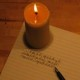## Mandelflug

A few days ago, I promised to write more about Mandelbulb, a three-dimensional fractal related to the Mandelbrot set. It has taken several days on a two-processor machine, but now the first movie is finally ready.

However, I would like to tell you a bit about sequences first. Sequences are, well, just numbers following one another; exactly what numbers make up the sequence is determined by certain rules.

Let us try to square numbers: 2, 4, 16, 256, 32768, ...  -- these numbers will grow very quickly. We might try to start with a smaller number instead: 1, 1, 1, ... -- I will admit this to be a rather boring sequence: 12=1, so there is little change. Maybe we could try this: 0.1, 0.01, 0.0001, ... -- now the numbers are actually shrinking. So: if we keep on squaring a number less than one in this way, it will shrink to nothing; numbers larger than one are growing, and one itself remains unchanged. By the way, trying negative number will not change this pattern -- the number will become positive during the first step, and the sequence proceeds as before. If we draw the number line, it might look a bit like this:Everything between -1 and +1 remains finite. All other numbers will grow ever larger when squared. We could try to make our formula (i.e. keep on squaring) more complicated, but we would not get funny pictures, anyway: the number line is, well, just a single line -- what can we make of it? Having an area to draw on would be much nicer:How shall we assign numbers to different spots on this plane? Luckily, this is no problem in mathematics: we simply invent new numbers. We shall do this in such a way that in addition to zero left of one and to to the right, we will have additional number above and below. Now we have to think of a way to square such numbers:We imagine a clock's hand pointing to each number. When trying to square such a number, we will first square its length. Then, we shall turn its hand a bit: one o'clock gets two o'clock, three o'clock becomes six o'clock, and four o'clock becomes eight o'clock. And, just in case you are wondering: mathematicians' clocks turn the wrong way -- and in this case, twelve o'clock (zero) is to the right, not on top.

This definition might look a tad strange, but it has a distinct advantage: all those ordinary numbers on the number line are at twelve o'clock (the positive ones), or six o'clock (the negative ones). When squaring them according to our new rule, the positive numbers will remain positive numbers, and the negative ones will be turned from six to twelve o'clock. That is, when squaring a number from the number line, we obtain a number from the positive half of the number line. Since we are squaring each hand's length, the result is just the same as when following the ordinary rules from school.Going back to sequences, we square away. The result is a bunch of hands rotating madly -- but all hands longer than on grow indefinitely, while the shorter ones shrink away to nothing.

This lets us draw at least a little picture, if not a very interesting one: a disc with radius one noting all numbers shrinking when they are squared.

It seems obvious that a more complex formula can create more complex pictures. However, if you think the formula has to be much more complex, you are mistaken: try to square and add the number alternately, like this: 12=1, 1+1=2, 22=4, 4+1=5, 52=25; thus: 1, 2, 4, 5, 25, ... and the numbers are getting ever larger. Starting with a negative number, we encounter a small surprise: -22=4, 4+(-2)=2, 22=4, 4+(-2)=2... we are getting an infinite sequence of the number two! Therefore, when painting every converging sequence black (i.e. every sequence remaining finite), we will not get a circle: +1 would remain white, but -2 would become black. Maybe an ellipse? It is hard to tell intuitively what our clock-hand numbers do, so we have to use a computer to calculate the picture. This is the result:It is surprising and quite wonderful that such a simple formula creates such a complex image; and this simplicity is, to a large extent, the beauty of the Mandelbrot set: one gets so much more than one has put in.

Finally, I would like to show my movie of the three-dimensional variant. An explanation of the corresponding formula will have to wait until next time. The fractal looks pretty smooth here, whereas the two-dimensional Mandelbrot set is quite hairy. This is not a property of the Mandelbulb, but of my alculations: in order to get a result in no more than a few days, I have calculated only the first two steps of the sequence. To get a more realistic picture, I would have had to use ten or so. Moreover, I will do something about the rough, pixelled structure.

Those of you who have paid very close attention might notice that while we have defined how to square a clock-hand number, we have neglected to define the summation. However, doing so is quite simple: to add to hands, we shift one of them so that its base coincides with the tip of the other hand. During that shift, neither hand must change the direction in which it points. The sum is then defined as a new hand that points from the base of the first to the tip of the second hand. This will look familiar if you know vectors.

Moreover, the clock-hand numbers will look quite familiar to those who know complex numbers.

11.34 pm 1 Kommentardeutsch

vonkirjoittaessani

### One Response to “Mandelflug”

1. kirjoittaessani » Blog Archive » Mandelpuzzle Says:

[...] einiger Zeit hatte ich angekündigt, für ein neues Bild der Mandelbulb eine weitere Iterationsstufe zu berechnen. Leider hat sich [...]